Courses

# MCQ (Previous Year Questions) - Chemical Equilibrium (Level 2)

## 7 Questions MCQ Test Chemistry for JEE Advanced | MCQ (Previous Year Questions) - Chemical Equilibrium (Level 2)

Description
This mock test of MCQ (Previous Year Questions) - Chemical Equilibrium (Level 2) for JEE helps you for every JEE entrance exam. This contains 7 Multiple Choice Questions for JEE MCQ (Previous Year Questions) - Chemical Equilibrium (Level 2) (mcq) to study with solutions a complete question bank. The solved questions answers in this MCQ (Previous Year Questions) - Chemical Equilibrium (Level 2) quiz give you a good mix of easy questions and tough questions. JEE students definitely take this MCQ (Previous Year Questions) - Chemical Equilibrium (Level 2) exercise for a better result in the exam. You can find other MCQ (Previous Year Questions) - Chemical Equilibrium (Level 2) extra questions, long questions & short questions for JEE on EduRev as well by searching above.
QUESTION: 1

Solution:
QUESTION: 2

Solution:
QUESTION: 3

### At constant temperature, the equilibrium constant (Kp) for the decomposition reaction. N2O4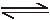2NO2 is expressed by Kp=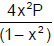where P is pressure, x is extent of decomposition. Which of the following statement is true ?     [JEE 2001]

Solution:
QUESTION: 4

Consider the following equilibrium in a closed container : N2O4(g)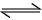2NO2(g).        [JEE 2002]

At a fixed temperature, the volume of the reaction container is halved. For this change, which of the following statements holds true regarding the equilibrium constant (KP) and degree of dissociation (α) :

Solution: For the equilibria: N2O4(g) ⟺ 2NO2(g) KP = KC x (RT)^ΔnSince temperature is constant so Kc or KP will remain constant. Further since volume ishalved, the pressure will be doubled so α will decrease so as to maintain the constancy of KC or KP.
QUESTION: 5

If Ag+ + NH3[Ag(NH3)]+ ; K1=1.6 × 10and

[Ag(NH3)+] + NH3[Ag(NH 3)+] ; K2=6.8 × 103

The formation constant of [Ag(NH3)2]+ is :                                   [JEE 2006]

Solution: Actually the second reaction has errorIt should be [Ag(NH3)]^+ +NH3--》[Ag(NH3)2]^+ and its k is given as 6.8×10^3So on adding both the reactions you will get the reaction as Ag^+ + 2NH3 --》[Ag(NH3)2]^+So the required formation constant is K1*k2 =1.6×6.8*10^6=1.088×10^7
*Multiple options can be correct
QUESTION: 6

The equilibrium

2CuI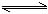Cuº + CuII

in aqueous medium at 25ºC shifts towards the left in the presence of       [JEE 2011]

Solution:
*Multiple options can be correct
QUESTION: 7

The thermal dissociation equilibrium of CaCO3 (s) is studied under different conditions.

CaCO3 (s)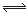CaO(s) + CO2(g)

For this equilibrium, the correct statement(s) is (are)           [JEE 2013]

Solution: